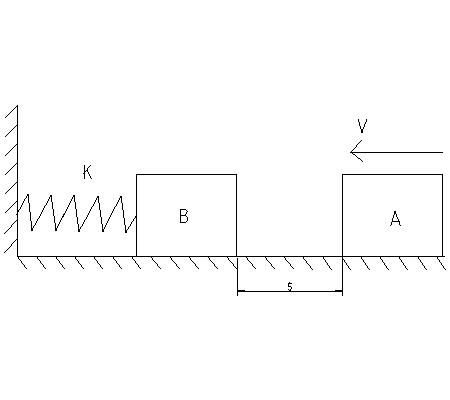The 18-kg block A slides on the surface for which ?k = 0.3. The block has a velocity v = 11 m/s...

Question:

The 18-kg block A slides on the surface for which ?k = 0.3. The block has a velocity v = 11 m/s when it is s = 3 m from the 12-kg block B. The unstretched spring has a stiffness k = 1000 N/m . Take e = 0.6. The coefficient of friction is the same for both blocks. (Figure 1) .Collision:

Collision is a phenomenon in which two or more than two bodies strike one another for a very short period of time. A collision may be elastic or inelastic. For a perfectly elastic collision the coefficient of restitution is 1, whereas for a perfectly inealstic collision, the coefficeint of restitution is zero.

Given data

• The mass of block A is: {eq}{m_A} = 18\;{\rm{kg}} {/eq}
• The mass of block B is: {eq}{m_B} = 12\;{\rm{kg}} {/eq}
• The velocity of block A is: {eq}v = 11\;{\rm{m/s}} {/eq}
• The stiffness of the spring is: {eq}k = 1100\;{\rm{N/m}} {/eq}
• The coefficient of friction is: {eq}{\mu _k} = 0.3 {/eq}
• The coefficient of restitution is: {eq}e = 0.6 {/eq}

The normal reaction on block A can be calculated as,

{eq}\begin{align*} N &= {m_A}g\\ &= 18\;{\rm{kg}} \times {\rm{9}}{\rm{.81}}\;{\rm{m/}}{{\rm{s}}^2}\\ N &= 176.58\;{\rm{N}} \end{align*} {/eq}

Here, the acceleration due to gravity is g.

The frictional force can be calculated as,

{eq}\begin{align*} f &= {\mu _k}N\\ & = 0.3 \times 176.58\;{\rm{N}}\\ f &= {\rm{52}}{\rm{.974}}\;{\rm{N}} \end{align*} {/eq}

Write the expression for the conservation of energy for block A.

{eq}\begin{align*} K{E_2} &= K{E_1} - {f_k} \times s\\ \dfrac{1}{2}{m_A}{{v'}_A}^2 &= \frac{1}{2}{m_A}{v^2} - {f_k} \times s \end{align*} {/eq}

Here, the kinetic energy of block A just before the collision is {eq}K{E_2} {/eq}, the initial kinetic energy of block A is {eq}K{E_1} {/eq}, the velocity of block A just before the impact is {eq}{v'_A} {/eq} and distance traveled by the block A is s.

Substitute the values in the above expression.

{eq}\begin{align*} \dfrac{1}{2} \times 18\;{\rm{kg}} \times \left( {{v'}_A}^2 \right) &= \dfrac{1}{2} \times 18\;{\rm{kg}} \times {\left( {11\;{\rm{m/s}}} \right)^2} - {\rm{52}}{\rm{.974}}\;{\rm{N}} \times 3\;{\rm{m}}\\ {{v'}_A} &= 10.16\;{\rm{m/s}} \end{align*} {/eq}

Write the expression for the coefficient of restitution.

{eq}e = \dfrac{{{{v''}_B} - {{v''}_A}}}{{{{v'}_A} - {{v'}_B}}} {/eq}

Here, the velocity of block B after the collision is {eq}{v''_B} {/eq}, the velocity of block A after the collision is {eq}{v''_A} {/eq}, the initial velocity of block B is {eq}{v'_B} {/eq}.

Substitute the values in the above expression.

{eq}\begin{align*} 0.6 &= \dfrac{{{{v''}_B} - {{v''}_A}}}{{10.16 - 0}}\\ {{v''}_B} - {{v''}_A} &= 6.0.96.........(1) \end{align*} {/eq}

Write the expression for the conservation of momentum.

{eq}{m_A}{v'_A} + {m_B}{v'_B} = {m_A}{v''_A} + {m_B}{v''_B} {/eq}

Substitute the values in the above expression.

{eq}\begin{align*} 18 \times 10.16 + 12 \times 0 &= 18{{v''}_A} + 12{{v''}_B}\\ 18{{v''}_A} + 12{{v''}_B} &= 182.88............(2) \end{align*} {/eq}

Solve equation (1) and equation (2).

{eq}\begin{align*} {{v''}_A} &= 3.6576\;{\rm{m/s}}\\ {{v''}_B} &= 9.7536\;{\rm{m/s}} \end{align*} {/eq}

Write the expression for the maximum compression of the spring.

{eq}\begin{align*} K{E_B} &= SE\\ \dfrac{1}{2}{m_B}{\left( {{{v''}_B}} \right)^2} &= \dfrac{1}{2}k{x^2}\\ x &= \sqrt {\dfrac{{{m_B}{{\left( {{{v''}_B}} \right)}^2}}}{k}} \end{align*} {/eq}

Here, the strain energy of the spring is SE.

Substitute the values in the above expression.

{eq}\begin{align*} x &= \sqrt {\dfrac{{12\;{\rm{kg}} \times {{\left( {9.7536\;{\rm{m/s}}} \right)}^2}}}{{1100\;{\rm{N/m}}}}} \\ x& = 1.018\;{\rm{m}} \end{align*} {/eq}

Thus, the maximum compression of the spring is {eq}1.018\;{\rm{m}} {/eq}.